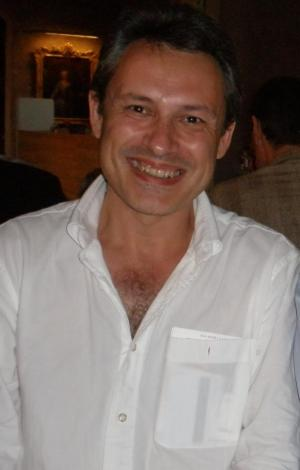Numerical Simulation of Flow in Deformable Porous Media
Speaker
Prof. Francisco José Gaspar
Department of Applied Mathematics, University of Zaragoza, Spain
Email: fjgaspar@unizar.es
Abstract

Here, we treat mathematical and practical aspects of poroelastic models, with an emphasis on their stable discretization and the highly efficient solution of the resulting algebraic systems, which are of saddle point type. Standard poroelastic models couple the equations of elastic deformation of a porous body with the effects of pressure from a fluid within the pores. However, this problem can interact with other different models to explain a more complex phenomenon describing more complicated multiphysics problems. Nowadays, poroelastic models are used to study problems in many diverse areas as geomechanics, hydrogeology, petrol engineering, biomechanics, etc. Therefore, the study of this type of problems is of great interest to scientists and engineers.